1:05 AM
Mechanical Similarity and the Virial Theorem

# Mechanical Similarity and the Virial Theorem

### Some Examples

Similar triangles are just scaled up (or down) versions of each other, meaning they have the same angles. Scaling means the same thing in a mechanical system: if a planet can go around the sun in a given elliptical orbit, another planet can go in a scaled up version of that ellipse (the sun remaining at the focus). But it will take longer: so we can't just scale the spatial dimensions, to get the same equation of motion we must scale time as well, and not in general by the same factor.

In fact, we can establish the relative scaling of space and time in this instance with very simple dimensional analysis. We know the planet's radial acceleration goes as the inverse square of the distance, so (radial acceleration)x(distance)2 = constant, the dimensionality of this expression is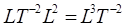, so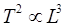, the square of the time of one orbit is proportional to the cube of the size of the orbit. A little more explicitly, the accelerationso for the same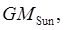if we double the orbit size, the equation will be the same but with orbital time up by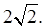Galileo established that real mechanical systems, such as a person, are not scale invariant. A giant ten times the linear dimensions of a human would break his hip on the first step. The point is that the weight would be up by a factor of 1,000, the bone strength, going as cross sectional area, only by 100.

Mechanical similarity is important is constructing small models of large systems. A particularly important application is to fluid flow, for example in assessing fluid drag forces on a moving ship, plane or car. There are two different types of fluid drag: viscous frictional drag, and inertial drag, the latter caused by the body having to deflect the medium as it moves through. The patterns of flow depend on the relative importance of these two drag forces, this dimensionless ratio, inertial/viscous, is called the Reynolds number. To give meaningful results, airflow speeds around models must be adjusted to give the model the same Reynolds number as the real system.

### Lagrangian Treatment

(Here we follow Landau.) Since the equations of motion are generated by minimizing the action, which is an integral of the Lagrangian along a trajectory, the motion won't be affected if the Lagrangian is multiplied by a constant. If the potential energy is a homogeneous function of the coordinates, rescaling would multiply it by a constant factor. If our system consists of particles interacting via such a potential energy, it will be possible to rescale time so that, rescaling both space and time, the Lagrangian is multiplied by an overall constant, so the equations of motion will look the same.

Specifically, if the potential energyis homogeneous of degree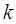, and the spatial coordinates are scaled by a factor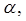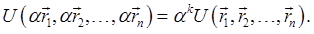To get the kinetic energy term to scale by the same factor, we take, so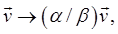the kinetic energy is scaled by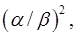for this to match the potential energy scaling, we must have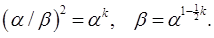For planetary orbits,, so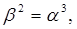confirming our hand waving derivation above.

For the simple harmonic oscillator,soand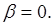What does that mean? Scaling up the orbit does not affect the time -- the oscillation time is always the same.

Falling under gravity: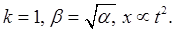So doubling the time scale requires quadrupling the length scale to get the scaled motion identical to the original.

### The Virial Theorem

For a potential energy homogeneous in the coordinates, of degreesay, and spatially bounded motion, there is a simple relation between the time averages of the kinetic energy,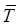, and potential energy,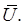It's called the virial theorem: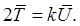Proof

Since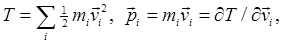we have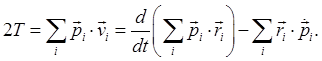We now average the terms in this equation over a very long time, that is, take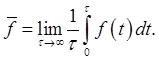Since we've said the orbits are bounded in space, and we assume also in momentum, the exact differential term contributes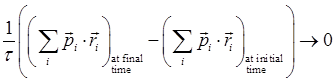in the limit of infinite time.

So we have the time averaged,

and for a potential energy a homogeneous function of degree k in the coordinates, from Euler's theorem:So, for example, in a simple harmonic oscillator the average kinetic energy equals the average potential energy, and for an inverse-square system, the average kinetic energy is half the average potential energy in magnitude, and of opposite sign (being of course positive).

Category: Education | Views: 309 | Added by: farrel | | Rating: 0.0/0